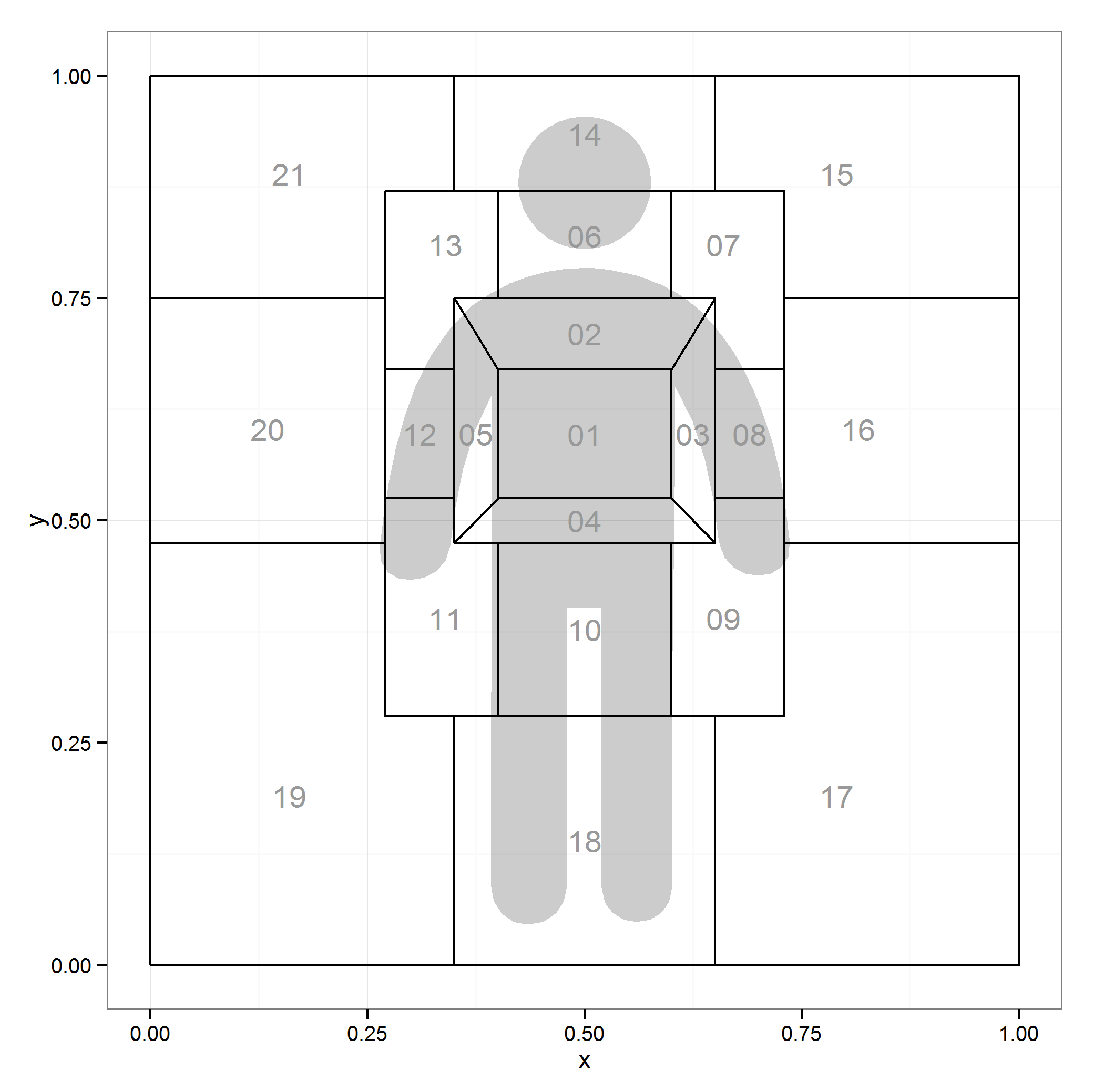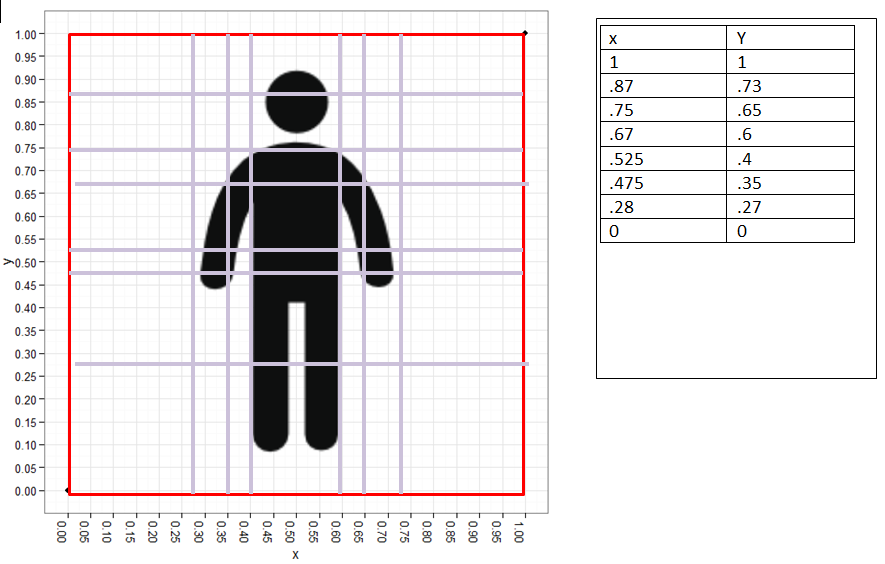## Shape File Selfies in ggplot2

In this post you will learn how to:

1. Create your own quasi-shape file
2. Plot your homemade quasi-shape file in `ggplot2`
3. Add an external svg/ps graphic to a plot
4. Change a `grid` grob's color and alpha

*Note get simple .md version here

## Background (See just code if you don't care much about the process)

I started my journey wanting to replicate a graphic called a space manikin by McNeil (2005) and fill areas in that graphic like a choropleth. I won't share the image from McNeil's book as it's his intellectual property but know that the graphic is from a gesturing book that divides the body up into zones (p. 275). To get a sense of what the manikin looks like here is the `ggplot2` version of it:Figure 1: ggplot2 Version of McNeil’s (2005) Space Manikin

While this is a map of areas of a body you can see where this could be extended to any number of spatial tasks such as mapping the layout of a room.

## 1. Creating a Quasi-Shape File

So I figured “zones” that's about like states on a map. I have toyed with choropleth maps of the US in the past and figured I'd generalize this learning. The difference is I'd have to make the shape file myself as the maps package doesn't seem to have McNeil’s space manikin.

Let's look at what `ggplot2` needs from the `maps` package:

```library(maps); library(ggplot2)
``````##     long   lat group order  region subregion
## 1 -87.46 30.39     1     1 alabama      <NA>
## 2 -87.48 30.37     1     2 alabama      <NA>
## 3 -87.53 30.37     1     3 alabama      <NA>
## 4 -87.53 30.33     1     4 alabama      <NA>
## 5 -87.57 30.33     1     5 alabama      <NA>
## 6 -87.59 30.33     1     6 alabama      <NA>
``````

Hmm coordinates, names of regions, and order to connect the coordinates. I figured I can handle that. I don't 100% know what a shape file is, mostly that it’s a file that makes shapes. What we're making may or may not technically be a shape file but know we're going to map shapes in `ggplot2` (I use the quasi to avoid the wrath of those who do know precisely what a shape file is).

I needed to make the zones around an image of a person so I first grabbed a free png silhouette from: http://www.flaticon.com/free-icon/standing-frontal-man-silhouette_10633. I then knew I'd need to add some lines and figure out the coordinates of the outlines of each cell. So I read the raster image into R, plotted in `ggplot2` and added lots of grid lines for good measure. Here's what I wound up with:

```library(png); library(grid); library(qdap)
url_dl(url="http://i.imgur.com/eZ76jcu.png")
file.rename("eZ76jcu.png", "body.png")
img <- rasterGrob(readPNG("body.png"), 0, 0, 1, 1, just=c("left","bottom"))
ggplot(data.frame(x=c(0, 1), y=c(0, 1)), aes(x=x, y=y)) +
geom_point() +
annotation_custom(img, 0, 1, 0, 1) +
scale_x_continuous(breaks=seq(0, 1, by=.05))+
scale_y_continuous(breaks=seq(0, 1, by=.05)) + theme_bw() +
theme(axis.text.x=element_text(angle = 90, hjust = 0, vjust=0))
```Figure 2: Silhouette from ggplot2 With Grid Lines

### 1b. Dirty Deeds Done Cheap

I needed to get reference lines on the plot so I could begin recording coordinates. Likely there's a better process but this is how I approached it and it worked. I exported the ggplot in Figure 2 into (GASP) Microsoft Word (I may have just lost a few die hard command line folks). I added lines there and and figured out the coordinates of the lines. It looked something like this:Figure 3: Silhouette from ggplot2 with MS Word Augmented Border Lines

After that I began the tedious task of figuring out the corners of each of the shapes (“zones”) in the space manikin. Using Figure 3 and a list structure in R I mapped each of the corners, the approximate shape centers, and the order to plot the coordinates in for each shape. This is the code for corners:

```library(qdap)
dat <- list(
`01`=data.frame(x=c(.4, .4, .6, .6), y=c(.67, .525, .525, .67)),
`02`=data.frame(x=c(.35, .4, .6, .65), y=c(.75, .67, .67, .75)),
`03`=data.frame(x=c(.6, .65, .65, .6), y=c(.525, .475, .75, .67)),
`04`=data.frame(x=c(.4, .35, .65, .6), y=c(.525, .475, .475, .525)),
`05`=data.frame(x=c(.35, .35, .4, .4), y=c(.75, .475, .525, .67)),
`06`=data.frame(x=c(.4, .4, .6, .6), y=c(.87, .75, .75, .87)),
`07`=data.frame(x=c(.6, .6, .65, .65, .73, .73), y=c(.87, .75, .75, .67, .67, .87)),
`08`=data.frame(x=c(.65, .65, .73, .73), y=c(.67, .525, .525, .67)),
`09`=data.frame(x=c(.6, .6, .73, .73, .65, .65), y=c(.475, .28, .28, .525, .525, .475)),
`10`=data.frame(x=c(.4, .4, .6, .6), y=c(.475, .28, .28, .475)),
`11`=data.frame(x=c(.27, .27, .4, .4, .35, .35), y=c(.525, .28, .28, .475, .475, .525)),
`12`=data.frame(x=c(.27, .27, .35, .35), y=c(.67, .525, .525, .67)),
`13`=data.frame(x=c(.27, .27, .35, .35, .4, .4), y=c(.87, .67, .67, .75, .75, .87)),
`14`=data.frame(x=c(.35, .35, .65, .65), y=c(1, .87, .87, 1)),
`15`=data.frame(x=c(.65, .65, .73, .73, 1, 1), y=c(1, .87, .87, .75, .75, 1)),
`16`=data.frame(x=c(.73, .73, 1, 1), y=c(.75, .475, .475, .75)),
`17`=data.frame(x=c(.65, .65, 1, 1, .73, .73), y=c(.28, 0, 0, .475, .475, .28)),
`18`=data.frame(x=c(.35, .35, .65, .65), y=c(.28, 0, 0, .28)),
`19`=data.frame(x=c(0, 0, .35, .35, .27, .27), y=c(.475, 0, 0, .28, .28, .475)),
`20`=data.frame(x=c(0, 0, .27, .27), y=c(.75, .475, .475, .75)),
`21`=data.frame(x=c(0, 0, .27, .27, .35, .35), y=c(1, .75, .75, .87, .87, 1))
)

dat <- lapply(dat, function(x) {
x\$order <- 1:nrow(x)
x
})

space.manikin.shape <- list_df2df(dat, "id")[, c(2, 3, 1, 4)]
```

And the code for the centers:

```centers <- data.frame(
id = unique(space.manikin.shape\$id),
center.x=c(.5, .5, .625, .5, .375, .5, .66, .69, .66, .5, .34, .31,
.34, .5, .79, .815, .79, .5, .16, .135, .16),
center.y=c(.597, .71, .5975, .5, .5975, .82, .81, .5975, .39, .3775, .39,
.5975, .81, .935, .89, .6025, .19, .14, .19, .6025, .89)
)
```

There you have it folks your very own quasi-shape file. Celebrate the fruits of your labor by plotting that bad Oscar.

``` ggplot(centers) + annotation_custom(img,0,1,0,1) +
geom_map(aes(map_id = id), map = space.manikin.shape, colour="black", fill=NA) +
theme_bw()+
expand_limits(space.manikin.shape) +
geom_text(data=centers, aes(center.x, center.y, label = id), color="grey60")
```Figure 4: Plotting the Quasi-Shape File and a Raster Image

Then I said I may want to tone down the color of the silhouette a bit so I can plot geoms atop without distraction. Here's that attempt.

```img[["raster"]][img[["raster"]] == "#0E0F0FFF"] <- "#E7E7E7"

ggplot(centers) + annotation_custom(img,0,1,0,1) +
geom_map(aes(map_id = id), map = space.manikin.shape, colour="black", fill=NA) +
theme_bw()+
expand_limits(space.manikin.shape) +
geom_text(data=centers, aes(center.x, center.y, label = id), color="grey60")
```Figure 5: Altered Raster Image Color

## 3. Add an External svg/ps

I realized quickly a raster was messy. I read up a bit on them in the R Journal (click here). In the process of reading and fooling around with Picasa I turned my original silhouette (body.png) blue and couldn't fix him. I headed back to http://www.flaticon.com/free-icon/standing-frontal-man-silhouette_10633 to download another. In this act I saw you could download a svg file of the silhouette. I thought maybe this will be less messier and easier to change colors. This led me to a google search and finding the `grImport` package after seeing this listserve post. And then I saw an article from Paul Murrell (2009) and figured I could turn the svg (I didn't realize what svg was until I opened it in Notepad++) into a ps file and read into R and convert to a flexible grid grob.

Probably there are numerous ways to convert an svg to a ps file but I chose a cloud convert service. After I read the file in with `grImport` per the Paul Murrell (2009) article. You're going to have to download the ps file HERE and get to your working directory.

```browseURL("https://github.com/trinker/space_manikin/raw/master/images/being.ps")
## Sorry I don't know how to automate this.
library(grImport)

## Convert to xml
PostScriptTrace("being.ps")

## Read back in and convert to a grob

## Plot it
ggplot(centers) + annotation_custom(being_img,0,1,0,1) +
geom_map(aes(map_id = id), map = space.manikin.shape,
colour="black", fill=NA) +
theme_bw()+
expand_limits(space.manikin.shape) +
geom_text(data=centers, aes(center.x, center.y,
label = id), color="grey60")
```Figure 6: Quasi-Shape File with Grob Image Rather than Raster

## 4. Change a `grid` Grob's Color and Alpha

Now we have a flexible grob we can mess around with colors and alpha until our heart's content.

`str` is our friend to figure out where and how to mess with the grob (`str(being_img)`). That leads me to the following changes to the image to adjust color and/or alpha (transparency).

```being_img[["children"]][][[c("gp", "fill")]] <-
being_img[["children"]][][[c("gp", "fill")]] <- "black"

being_img[["children"]][][[c("gp", "alpha")]] <-
being_img[["children"]][][[c("gp", "alpha")]] <- .2

## Plot it
ggplot(centers) + annotation_custom(being_img,0,1,0,1) +
geom_map(aes(map_id = id), map = space.manikin.shape,
colour="black", fill=NA) +
theme_bw()+
expand_limits(space.manikin.shape) +
geom_text(data=centers, aes(center.x, center.y,
label = id), color="grey60")
```Figure 7: Quasi-Shape File with Grob Image Alpha = .2

## Let's Have Some Fun

Let's make it into a choropleth and a density plot. We'll make some fake fill values to fill with.

```set.seed(10)
centers[, "Frequency"] <- rnorm(nrow(centers))

being_img[["children"]][][[c("gp", "alpha")]] <-
being_img[["children"]][][[c("gp", "alpha")]] <- .25

ggplot(centers, aes(fill=Frequency)) +
geom_map(aes(map_id = id), map = space.manikin.shape,
colour="black") +
theme_bw()+
expand_limits(space.manikin.shape) +
geom_text(data=centers, aes(center.x, center.y,
label = id), color="black") +
annotation_custom(being_img,0,1,0,1)
```Figure 8: Quasi-Shape File as a Choropleth

```set.seed(10)
centers[, "Frequency2"] <- sample(seq(10, 150, by=20, ), nrow(centers), TRUE)

centers2 <- centers[rep(1:nrow(centers), centers[, "Frequency2"]), ]

ggplot(centers2) +
#       geom_map(aes(map_id = id), map = space.manikin.shape,
#       colour="grey65", fill="white") +
stat_density2d(data = centers2,
aes(x=center.x, y=center.y, alpha=..level..,
fill=..level..), size=2, bins=12, geom="polygon") +
scale_fill_gradient(low = "yellow", high = "red") +
scale_alpha(range = c(0.00, 0.5), guide = FALSE) +
theme_bw()+
expand_limits(space.manikin.shape) +
geom_text(data=centers, aes(center.x, center.y,
label = id), color="black") +
annotation_custom(being_img,0,1,0,1) +
geom_density2d(data = centers2, aes(x=center.x,
y=center.y), colour="black", bins=8, show_guide=FALSE)
```Figure 9: Quasi-Shape File as a Density Plot

Good times were had by all.

Created using the reports (Rinker, 2013) package

Get the .Rmd file here

## References